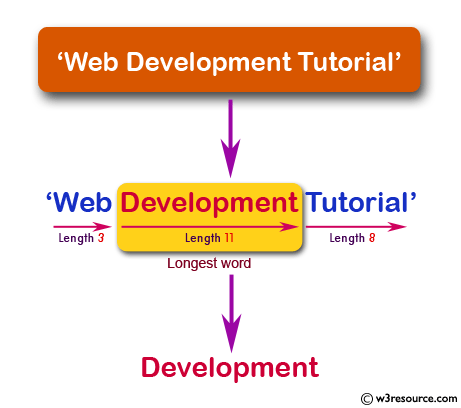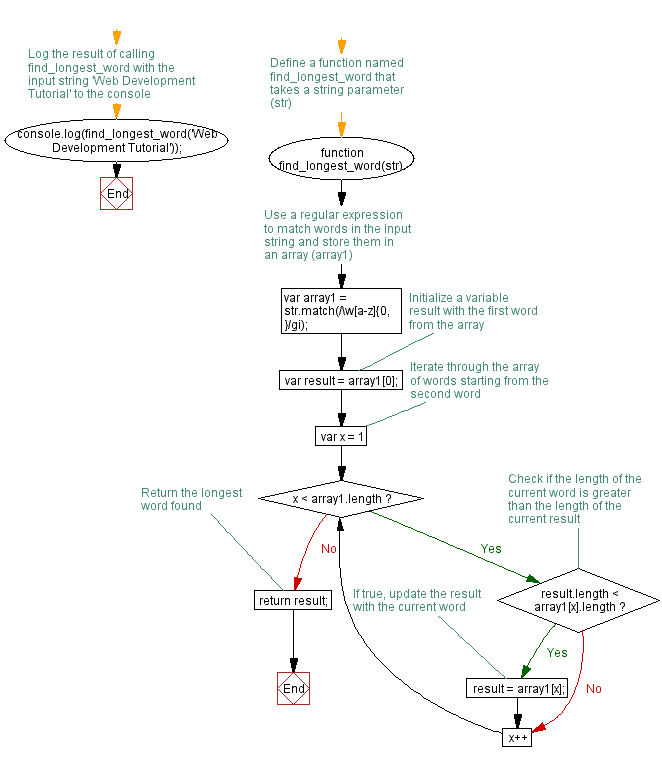# JavaScript: Find the longest word within a string

## JavaScript Function: Exercise-6 with Solution

Write a JavaScript function that accepts a string as a parameter and find the longest word within the string.
Sample Data and output:
Example string: 'Web Development Tutorial'
Expected Output: 'Development'

Pictorial Presentation:Sample Solution: -

HTML Code:

``````<!DOCTYPE html>
<html>
<meta charset=utf-8 />
<title>Find the longest word within a string</title>
<body>

</body>
</html>
```
```

JavaScript Code:

``````function find_longest_word(str)
{
var array1 = str.match(/\w[a-z]{0,}/gi);
var result = array1;

for(var x = 1 ; x < array1.length ; x++)
{
if(result.length < array1[x].length)
{
result = array1[x];
}
}
return result;
}
console.log(find_longest_word('Web Development Tutorial'));
```
```

Sample Output:

```Development
```

Explanation:
Assume str = '@Web Development #Tutorial';

The match() method is used to retrieve the matches when matching a string against a regular expression.
Therefore str.match(/\w[a-z]{0,}/gi) will return ["Web", "Development", "Tutorial"].

For loop checks the length of the array element and compare with previous one and finally return the longest string.

The length property represents an unsigned, 32-bit integer that is always numerically greater than the highest index in the array.
Syntax -> arr.length

Flowchart:Live Demo:

See the Pen JavaScript -Converts the first letter of each word of a string in upper case-function-ex- 5 by w3resource (@w3resource) on CodePen.

Improve this sample solution and post your code through Disqus

What is the difficulty level of this exercise?

﻿

## JavaScript: Tips of the Day

Returns 1 if the array is sorted in ascending order, -1 if it is sorted in descending order or 0 if it is not sorted

Example:

```const tips_Sorted = arr => {
let direction = -(arr - arr);
for (let [i, val] of arr.entries()) {
direction = !direction ? -(arr[i - 1] - arr[i]) : direction;
if (i === arr.length - 1) return !direction ? 0 : direction / Math.abs(direction);
else if ((val - arr[i + 1]) * direction > 0) return 0;
}
};
console.log(tips_Sorted([0, 1, 2, 2]));
console.log(tips_Sorted([5, 7, 3]));
console.log(tips_Sorted([8, 6, 4]));
```

Output:

```1
0
-1
```